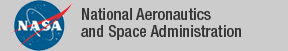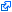### Top File of PVS groups Library

```
% Andre Luiz Galdino
% Federal University of Goais, Brazil
%
%
% The following theorem of group theory are formalized:
%
% - Cauchy Theorem;
% - Isomorphism Theorems;
% - Burside Theorem;
% - Sylow Theorems;
% - New version of the Lagrange Theorem;
% - A variation of the fundamental principle of counting;
% - A variation of the formula for permutation with repetition;
% - Group Action, stabilizer, orbit, normalizer, centralizer, index of a
%   subgroup in a group, and properties;
% - Class Equation;
% - p-groups and properties;
% - Product of Subgroups;
% - New version of the Zn Group, left (right) cosets, factor group and
%   properties.

top[T: TYPE, *: [T,T -> T], one: T]: THEORY

BEGIN
ASSUMING IMPORTING algebra@group_def

T_is_group: ASSUMPTION group?[T,*,one](fullset[T])

ENDASSUMING

IMPORTING sylow_theorems[T, *, one]

END top
```
The tagidentifies links that are outside the NASA domain